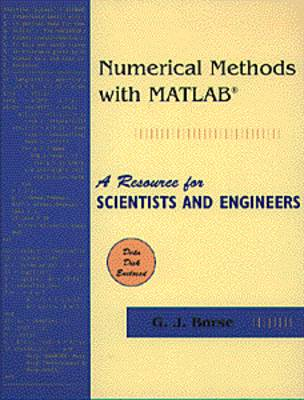•# Numerical Methods Using MATLAB (Hardback)

(author)
£35.99
Hardback Published: 13/12/1996

This product is currently unavailable.

NUMERICAL METHODS WITH MATLAB- is intended as a resource or compendium of methods suitable for use in numerical methods and engineering problem-solving courses. This book details the main topics of numerical methods, such as linear analysis, analysis of functions of one or several variables, data analysis and modeling, and the solution of ordinary and partial differential equations. Borse emphasizes practical applications, while providing sufficient theory for students to understand the underlying operation, and strengths and weaknesses, of each technique. MATLAB- is used throughout; the text works equally well for students with or without prior background in MATLAB-. Students inexperienced in the use of MATLAB- can refer to the MATLAB- programming appendix at the back of the book, which introduces MATLAB- topics in the same sequence as they are presented in the numerical methods sections of the text. A computer disk containing a MATLAB- tutorial accompanies the text."

Publisher: Cengage Learning, Inc
ISBN: 9780534938222
Weight: 1202 g
Dimensions: 248 x 197 x 31 mm